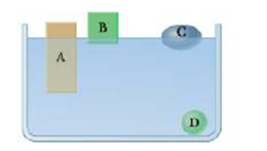Chapter 9, Problem 3CQ

Chapter
Section
Textbook Problem

Four solid, uniform objects are placed in a container of water (Fig. CQ9.3) Rank their densities from highest to lowest.Figure CQ9.3

To determine

The densities of the objects.

Explanation

When an object floats, its weight is equal to the buoyant force acting on it. Therefore,

ρogVo=ρwgVsub

• ρw is the density of water.
• ρo is the density of object.
• g is the acceleration due to gravity.
• Vo is the volume of the object submerged.
• Vsub is the volume of the object submerged.

Rewrite the equation in terms of ρo

Still sussing out bartleby?

Check out a sample textbook solution.

See a sample solution

The Solution to Your Study Problems

Bartleby provides explanations to thousands of textbook problems written by our experts, many with advanced degrees!

Get Started